## Monday, July 29, 2013

### TI-84+ Color Silver Edition: MathPrint versus ClassicPrint

I have stated that the best way to use programs on the TI-84+ C Silver Edition (and previous editions) that the most efficient way to run programs is in Classic Mode (one-line mode).

This especially comes into play when repetitive loops, like For loops, are involved.

A sample program is shown below. I ran each test twice.

PROGRAM:TEST900
ClrHome
For(I,1,500)
Output(1,1,I)
End
Output(2,1,"DONE")

Times:
TEST900 run in CLASSIC Mode: 7.5 sec, 7.81 sec
TEST900 run in MATHPRINT Mode: 8.75 sec, 9.25 sec

But what if we want to always operate in MATHPRINT mode? How about if we switch to CLASSIC at the beginning of the program and switch back to MATHPRINT in the end? How is the run-time affected?

PROGRAM:TEST900
CLASSIC
ClrHome
For(I,1,500)
Output(1,1,I)
End
Output(2,1,"DONE")
MATHPRINT

The times I got were 9.62 seconds and then 10 seconds. So, as far as this test program is concerned, switching between CLASSIC and MATHPRINT modes does not help.

Disclaimer: This is only one program - and I did this test out of pure curiosity.

Enjoy!

Eddie

## Sunday, July 28, 2013

### Discounts and Sales Tax: What is the Final Price?

SALE! GET THIS WONDERFUL ITEM FOR A SUBSTANTIAL DISCOUNT! GOOD FOR THIS WEEKEND ONLY!

Chances are that we have all seen this advertisement at least one time in our lives. Chances are that if you are shopping with someone, that person asks what the price is after the discount. And in a lot of instances, depending on where you live, you have to take sales tax into consideration. Today's blog is about how to find the final price after the discount and sales tax.

Note: All the examples presented here are using prices stated in dollars (\$). Rest assured the concepts hold for all other units of currency. Also, all dollar amounts are rounded to two decimal places.

The Math Behind it All

Discounts

Let D be the discount. Since the discount is customarily stated as a percentage, such as "take 20% off of everything on this rack", we'll express the discount as its decimal equivalent: 0.01*D. Discounts are subtracted from the price.

For example, a item normally sold for \$59.99 which has a 20% discount has a discounted price of \$47.99.

\$59.99 - 20%
\$59.99 - \$59.99 * 0.01 * 20
\$59.99 * (1 - 0.20)
\$59.99 * 0.80
\$47.99

A general formula for subtracting D% from X is:

X - D%
X - X * 0.01 * D
X * (1 - 0.01 * D)

Sales Tax

Let S be the sales tax. Sales tax works like discounts, except that sales tax is added to the price. We'll express the sales tax as its decimal equivalent: 0.01*S.

Where I live, which is located in the County of Los Angeles in California, the sales tax is 9%. For example, if I purchase a taxable item for \$59.99, taking sales tax into account, the total cost to me is \$65.39.

\$59.99 + 9%
\$59.99 + \$59.99 * 0.01 * 9
\$59.99 + \$5.40
\$65.39

Likewise, we can use a general formula for adding S% to X is:

X + S%
X + 0.01 * S * X
X * (1 + 0.01 * S)

The Final Price of a Discounted Item After Sales Tax

Let's come up with a general formula where we determine the final price of an item which normally sells for \$P (or whatever currency you work with), sold at a discount D%, which the item subject to sales tax of S%.

Customarily, the discount gets applied before the sales tax. Then the final price works out to be:

\$P - D% + S%

(\$P - D%) + S%
(\$P - \$P * 0.01 * D) + S%
(\$P * (1 - 0.01 * D) + S%

(\$P * (1 - 0.01 * D) + (\$P * (1 - 0.01 * D)) * S * 0.01
(\$P * (1 - 0.01*D))(1 + 0.01*S)

So the final cost is:
\$P * (1 - 0.01*D) * (1 + 0.01*S)

Taking from our example before, let's say that the item originally priced at \$59.99 is discounted at 20%. What is the final cost after 9% sales tax is applied?

P = \$59.99
D = 20
S = 9
And the final cost is:

\$59.99 * (1 - 0.01*20) * (1 + 0.01*9) = \$52.31

In the next section, we will determine a "combined rate".

Simplified Rate Considering Both Discount and Sales Tax

Here we are going to find a general formula for a "combined rate", or a rate that takes both the discount and sales tax rate into account.

Basically, the "combined rate" is what the final price would be if the original price was \$1.00. (\$P = \$1.00)

The combined rate is (1 - 0.01D)*(1+0.01S)

In our example the combined rate for an item discounted 20%, subject to sales tax of 9% is 0.872. (87.2%)

(1 - 0.01*20)(1 + 0.01*9)
0.872

Under these conditions, you should expect to pay 87.2% of the original price when it is all said and done. For an item with an original price of \$59.99, the final cost is:

Price * Combined Rate
\$59.99 * 0.872
\$52.31

Another example: item has an original price of \$18.99. The discount is 10% while the sales tax rate is 9%

Combined rate:
(1 - 0.10 * 10) * (1 + 0.01 * 9)
0.981

Final cost:
\$18.99 * 0.981
\$18.63

As a check, let's find the final cost if we applied the discount then sales tax step by step.
(\$18.99 - 10%) + 9%
(\$18.99 - \$18.99 * 0.01 * 10) + 9%
\$17.09 + 9%
\$17.09 + \$17.09 * 0.01 * 9
\$18.63

It checks out.

This is a quick table of combined rates using various sales tax and discount rates. The iOS App Numbers is used to generate this table.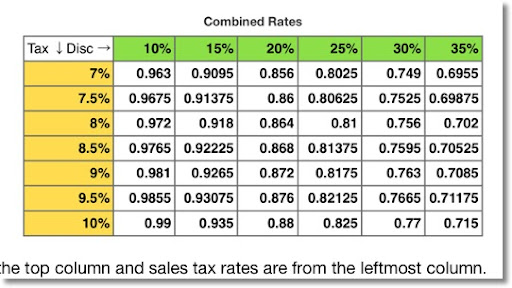Example: What is the final cost for at item originally priced \$69.95 that is discounted 35%. The sales tax rate is 10%.

From the table above, the combined rate is 0.715. The final cost is \$69.95 * 0.715 = \$50.01.

I want to do another mathematical series. I plan to do a short series of ordinary differential equations within the next couple of months, but if there is anything you suggest, subjects, calculators, calculator programming languages, please do. Many thanks as always.

My next blog entry will be within a couple of weeks. I am taking care of family and also going on a short vacation next weekend - hopefully the weather at Landers, CA will be nice as I go on my first astronomical star party.

Eddie

This blog is property of Edward Shore. 2013

## Tuesday, July 23, 2013

### Roots of x^A = 0 and x^A + x^B = 0

In today's blog, we will find the roots to:

(1) x^A = 0, and
(2) x^A + x^B = 0

In the following, let A and B be any constants.

x^A = 0

One logical way to approach this is take the logarithm of both sides, which leads to

ln(x^A) = ln 0

However, ln 0 is undefined.

How about a more direct route?

x^A = 0
(x^A)^(1/A) = 0^(1/A)
x = 0^(1/A)

Whether 0^(1/A) has a value depends on the value of A itself. If A>0, then 0^(1/A) = 0.

However, if A = 0, 1/0 is undefined and therefore 0^(1/0) is undefined.

If A<0, then 1/A < 0, -1/A > 0, and
0^(1/A) = 1/(1^(-1/A), which is undefined.

So x^A = 0 has a root only when A>0.

x^A + x^B = 0

Let's make two assumptions:
1. A and B are both positive, that is, A>0 and B>0.
2. B > A.

Using assumption 2, let B = A + n. (n = B - A). Then:

x^A + x^B = 0
x^A + x^(A + n) = 0
x^A + x^A * x^n = 0
x^A * (1 + x^n) = 0

This implies that either x^A = 0 or (1 + x^n) = 0

Case 1: x^A = 0.

Since we assumed that A>0, then the root of x^A = 0 is x = 0.

Case 2: 1 + x^n = 0

Then x^n = -1

If n is an integer, then the roots of -1 can be found by the formula:

(-1)^(1/n) = cos((2*k+1)*π/n) + i*sin((2*k+1)*π/n) for k
for k=0,1,2,...,n-1

(Source: Zwillinger, Daniel. CRC Standard Mathematical Tables and Formulae, 32nd Edition. CRC Press, 2012)

The roots to x^A + x^B = 0 with A > 0, B > 0, and B > A are:

x = 0, (-1)^(B - A)

Examples of x^A + x^B = 0

x^2 + x^4 = 0

x^2 + x^4 = 0
x^2 * (1 + x^2) = 0

x^2 = 0, x = 0
1 + x^2 = 0, x^2 = -1, x = i or x = -i. (Where i = √-1)

The roots are x = 0, x = i, and x = -i

x^2 + x^3 = 0

x^2 + x^3 = 0
x^2 * (1 + x) = 0

x^2 = 0, x = 0
1 + x = 0, x = -1

The roots are x = 0 and x = -1.

x^3 + x^5 = 0

x^3 + x^5 = 0
x^3 * (1 + x^2) = 0

Which implies that x^3 = 0 and 1 + x^2 = 0.

The roots are x = 0, x = i, and x = -i

Until next time everyone, good wishes,

Eddie

This blog is property of Edward Shore. 2013

## Friday, July 19, 2013

### Do A^x = 0 and A^x + B^x = 0 have roots?

In this blog entry we will look at two equations and see if there are solutions to them.

Things to Know:
In the realm of complex numbers, i = √(-1).

For the function f(x) = ln x:

If x > 0 and x is real, then ln x is also real and ln x = ln x.

If x < 0 and x is real, then ln x is complex and ln x = ln |x| + i * π.

If x is a complex number, such that x = a + bi, then:
ln x = ln r + i * θ where:
r = √(a^2 + b^2)
θ = atan(b/a) where -π ≤ θ ≤ π

Laws of Logarithm Needed:

Where a and b are any numbers:
ln (a^b) = b * ln a
ln(a * b) = ln a + ln b
ln(a / b) = ln a - ln b

And (a + b*i)^n = r^n * e^(i * π * θ) = r^n * (cos(n*θ) + i * sin(n*θ))

A^x = 0

(I) A^x = 0

Start by taking the logarithm of both sides:

(II) ln (A^x) = ln 0
(III) x * ln A = ln 0

And theoretically,

(IV) x = ln 0/ln A

Immediately we see a problem. The expression ln 0 is undefined. The function ln x approaches negative infinity when x approaches zero from the right side (that is x > 0 and calculating ln x as x is decreasing).

However, when x approaches from the left side, ln x approaches -infinity + i * π. There is no complex number makes the statement A^x = 0 true.

Therefore, A^x has no solutions. There is no x that makes statement (IV) in this section true.

A^x + B^x = 0

Let's see if we can get a closed form solution for x.

(I) A^x + B^x = 0

Assume that B can be rewritten as B = A^n., where n is any power. Then:

(II) A^x + (A^n)^x = 0
(III) A^x + (A^x)^n = 0

Factoring out A^x:

(IV) A^x * (1 + (A^x)^(n-1) ) = 0

From (IV) either:
A^x = 0, which can not happen sense from the previous section, A^x has no roots, real or complex.

So we have to look at the other possibility: (1 + (A^x)^(n-1) ) = 0.

Then:
(V) (A^x)^(n-1) = -1
(VI) A^x = -1^(1/(n-1))

The polar representation for -1 + 0i is 1*e^(i*π), a general solution for (VI) is:

(VII) A^x = cos((n-1) * π)+ i * sin((n-1)*π)

If n is an integer, then the roots of -1 can be found by the formula:

(-1)^(1/n) = cos((2*k+1)*π/n) + i*sin((2*k+1)*π/n) for k
for k=0,1,2,...,n-1

(Source: Zwillinger, Daniel. CRC Standard Mathematical Tables and Formulae, 32nd Edition. CRC Press, 2012)

From (VII) of this section, let α = cos((n-1) * π) and β = sin((n-1)*π) and

(VIII) A^x = α + i * β
(IX) ln A^x = ln (α + i * β)
(x) x * ln A = ln(α + i * β)

Hence, a general solution to A^x + B^x = 0 where B = A^n is:

(XI) x = ln(α + i * β)/ln A
Where α = cos((n-1) * π) and β = sin((n-1)*π)

There are no real solutions to A^x + B^x = 0, only complex ones.

An Example
2^x + 8^x = 0

Since 8 = 2^3,

2^x + (2^3)^x = 0
2^x + (2^x)^3 = 0
2^x * (1 + (2^x)^2) = 0

There is no solution for 2^x = 0, so we look to (1 + (2^x)^2) = 0.

(1 + (2^x)^2) = 0
(2^x)^2 = -1

The square roots of -1 are i and -i.

One solution is:
2^x = i
x * ln 2 = ln i
x = ln i / ln 2 ≈ 2.266180071i

The other is:
2^x = -i
x * ln 2 = ln -i
x = ln -i / ln 2 ≈ -2.266180071i

And that is a way to find the roots of A^x + B^x = 0.

Until next time, Eddie

This blog is property of Edward Shore. 2013

## Wednesday, July 17, 2013

### Solving 2^x + 4^x = A

Solve for x:

(I) 2^x + 4^x = A

Let A be any constant.

Then:

Note that 4=2^2. Then 4^x = (2^2)^x = (2^x)^2. Therefore:

(II) 2^x + (2^x)^2 = A

Let u = 2^x.

(III) u + u^2 = A

(IV) u^2 + u - A = 0

Solving the polynomial yields:

(V) u = (-1 ± √(1 + 4*A))/2

With u = 2^x:

(VI) 2^x = (-1 ± √(1 + 4*A))/2

Taking the logarithm of both sides, and with ln(B^C) = C ln B:

(VII) x * ln 2 = ln [ (-1 ± √(1 + 4*A))/2 ]

With ln(B/C) = ln B - ln C

(VIII) x * ln 2 = ln (-1 ± √(1 + 4*A) - ln 2

Solving for x:

(IX) x = [ ln (-1 ± √(1 + 4*A) ] / [ln 2] - 1

A General technique to solving A^x + B^x = C: (assuming B > A)

Let B = A^n. Then:

A^x + (A^x)^n = C

Let u = A^x, then solve the polynomial for u:

u + u^n - C = 0

Once the roots of u are found, then x = ln u/ln n

Solving this equation analytically is best when A, B, and C work "nicely".

Take care everyone, hope this helps and as always appreciate all the feedback!

Eddie

This blog is property of Edward Shore. 2013

## Friday, July 12, 2013

### At the Gas Pump: Use the ATM or Use Card to Pay Higher Gas Price?

Greetings everyone!

Gas Prices

As we go along our travels, we may see a gas station that offers to prices: the price per gallon if we use our ATM card or credit card, and a discounted price (usually a 10¢ discount) if we pay by cash.

But how much are we really saving - especially if we have use the ATM inside the gas station? (Let's assume that we are not fortunate enough to have the cash on hand before we arrive at the station.)

For example: The Corner Gas Station is has gas priced at \$3.95/gallon. However, if I pay by cash, Corner Gas will charge me only \$3.85/gallon. This sounds good but I have to use the ATM that will charge me a \$3.00 bank fee.

Suppose that I still want to pay the lower price, so I bite the bullet to take out the necessary cash. How many gallons do I have to buy to realize any true savings?

Thankfully some simple algebra can be used to provide the answer.

Let x be the number of gallons.

Then the total cost I pay if I use my card to pay is 3.95 * x.

The total cost I pay if use the ATM and pay cash is 3.85 * x + 3.00.

Equating the two costs:
3.95 * x = 3.85 * x + 3.00
0.10 * x = 3.00
x = 30

I would have to buy 30 gallons, for a total cost of \$118.50, for the costs to be equal, so basically I would have to drive a truck with a huge tank. My car only carries 8.5 gallons. What would be my costs using two options. (Again, assume I didn't bring the needed cash in advance)

Pay by card: 3.95 * 8.5 = 33.575 (\$33.58)
Pay by cash but also use the gas station's ATM: 3.85 * 8.5 + 3.00 = 35.725 (\$35.73)

When considering the hefty \$3.00 bank fee, I would be paying more to use the cash price.

Next time when I am in this situation, I won't act in such in rush to use the fee-loaded ATM. And to those who are using the no-fee-ever credit union cards at any ATM, consider yourself the smart one.

A General Formula

The number of gallons needed to buy to equalize the total cost between using a card and using cash but also using the gas station's ATM is:

x = ATM Fees / (card price - cash price)

Assumptions:
1. The card price is higher than the cash price.
2. You don't have the ample cash - for whatever reason.

Hope this helps someone save some money at the gas station.

Until next time,

Eddie

This blog is property of Edward Shore. 2013

## Wednesday, July 10, 2013

### x^3+ y^3 + z^3 = n^2

The goal is to find solutions of x^3+ y^3 + z^3 = n^2 where x, y, z, and n are integers greater than 1.

Using the following program to find solutions (TI nSpire App):

Define test32(k,n)=
Prgm
:x2:={}
:y2:={}
:z2:={}
:n2:={}
:For a,k,n
:For b,k,n
:For c,k,n
:d:=(a^(3)+b^(3)+c^(3))^(1/2)
:If fPart(d)=0 Then
:x2:=augment(x2,{a})
:y2:=augment(y2,{b})
:z2:=augment(z2,{c})
:n2:=augment(n2,{d})
:EndIf
:EndFor
:EndFor
:EndFor
:EndPrgm

Some solutions for testing integers from 1 to 50:
1^3 + 2^3 + 6^3 = 225 = 15^2
1^3 + 4^3 + 14^3 = 2809 = 53^2
2^3 + 12^3 + 50^3 = 126,736 = 356^2
3^3 + 3^3 + 3^3 = 81 = 9^2
4^3 + 4^3 + 17^3 = 5041 = 71^2
4^3 + 17^3 + 22^3 = 15,625 = 125^2
8^3 + 4^3 + 12^3 = 2304 = 48^2
8^3 + 13^3 + 15^3 = 6084 = 78^2
8^3 + 30^3 + 49^3 = 145,161 = 381^2
10^3 + 10^3 + 20^3 = 10,000 = 100^2
10^3 + 24^3 + 26^3 = 32,400 = 180^2
12^3 + 12^3 + 12^3 = 5184 = 72^2

In the range of 1 ≤ x ≤ 25, 1 ≤ y ≤ 25, and 1 ≤ z ≤ 25, there are 122 solutions.

In the range of 1 ≤ x ≤ 50, 1 ≤ y ≤ 50, and 1 ≤ z ≤ 50, there are 415 solutions. Presented next is a graph of √(x^3 + y^3 + z^3) and where square root of the sums stack up: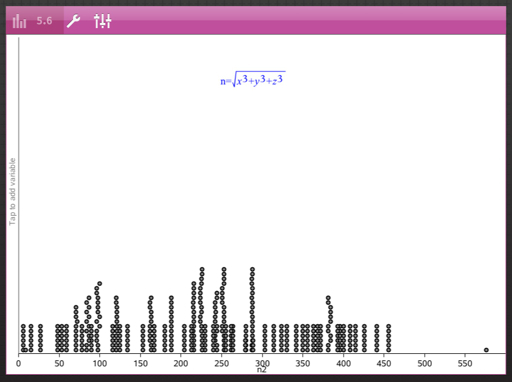Finally, using the MathStudio app, is a plot of solutions for 0 ≤ x ≤ 25, 0 ≤ y ≤ 25, and 0 ≤ z ≤ 25.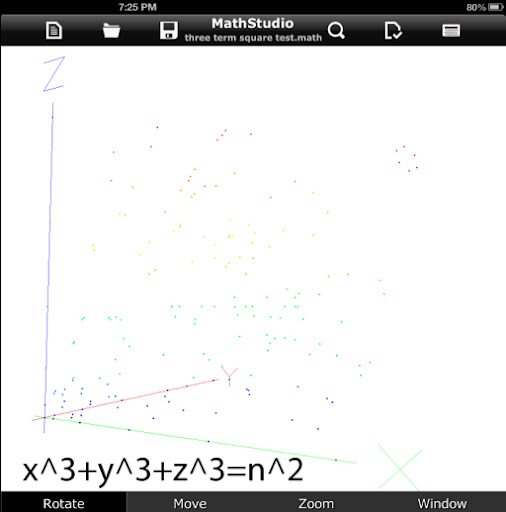Until next time,

Eddie

### x^2 + y^2 + z^2 = n^2

The goal is to find solutions of x^2 + y^2 + z^2 = n^2 where x, y, z, and n are integers greater than 1.

Using the following program to find solutions (TI nSpire App):

Define test22(n)=
Prgm
:x1:={}
:y1:={}
:z1:={}
:n1:={}
:For a,1,n
:For b,1,n
:For c,1,n
:d:=√(a^(2)+b^(2)+c^(2))
:If fPart(d)=0 Then
: x1:=augment(x1,{a})
: y1:=augment(y1,{b})
: z1:=augment(z1,{c})
: n1:=augment(n1,{d})
:EndIf
:EndFor
:EndFor
:EndFor
:EndPrgm

Some solutions for testing integers from 1 to 50:
1^2 + 8^2 + 32^2 = 1089 = 33^2
2^2 + 2^2 + 1^2 = 9 = 3^2
2^2 + 12^2 + 36^2 = 1444 = 38^2
3^2 + 6^2 + 6^2 = 81 = 9^2
4^2 + 7^2 + 32^2 = 1089 = 33^2
4^2 + 22^2 + 20^2 = 900 = 30^2
5^2 + 40^2 + 20^2 = 2025 = 45^2
6^2 + 2^2 + 9^2 = 121 = 11^2
6^2 + 10^2 + 15^2 = 361 = 19^2
6^2 + 42^2 + 7^2 = 1849 = 43^2
7^2 + 30^2 + 30^2 = 1849 = 43^2
8^2 + 5^2 + 44^2 = 2025 = 45^2
8^2 + 24^2 + 27^2 = 1369 = 37^2
9^2 + 30^2 + 50^2 = 3481 = 59^2

In the range of 1 ≤ x ≤ 25, 1 ≤ y ≤ 25, and 1 ≤ z ≤ 25, there are 288 solutions.

In the range of 1 ≤ x ≤ 50, 1 ≤ y ≤ 50, and 1 ≤ z ≤ 50, there are 1209 solutions. Presented next is a graph of √(x^2 + y^2 + z^2) and where square root of the sums stack up: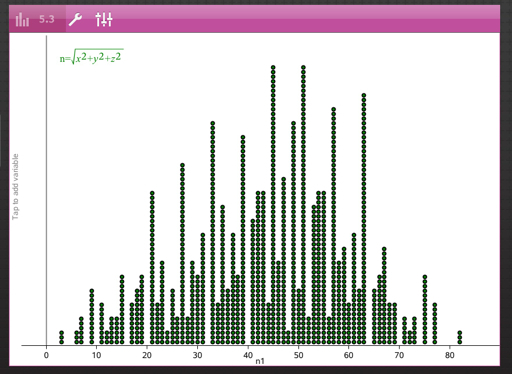Note that are two results (n) that show up the most.

For the range of 1 to 25, the dominating n's are 21 and 23, each resulting showing 33 times in the 288 solutions.

However, the most dominated results shifts as the range increases. For the range of 1 to 50, the dominating results are 45 and 51, each showing up 60 times in the 1209 solutions.

Finally, using the MathStudio app, is a plot of solutions for 0 ≤ x ≤ 25, 0 ≤ y ≤ 25, and 0 ≤ z ≤ 25.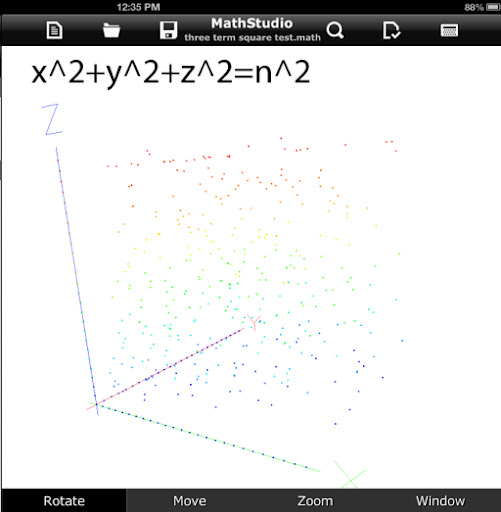Until next time,

Eddie

This blog is property of Edward Shore. 2013

## Friday, July 5, 2013

### Spherical Triangle Solver - TI-84+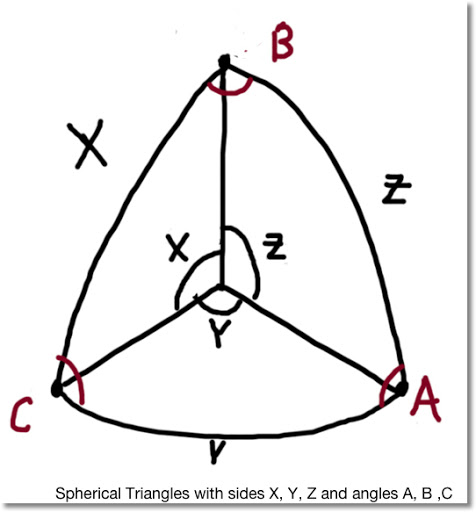Spherical Triangle Solver: Programmed on a TI-84+ C Silver Edition

This could easily be ported to other programming calculators given how basic the TI-84+ programming is.

Variables:
"Sides" (Subtending Angles): X, Y, Z
Corresponding Angles: A, B, C

I try to capture all possible traps. No guarantees by I try. This program is rather large, about 1,500 bytes. Ending quotes and parenthesis can be left out on the TI-84+ but I present them in the text for completeness.

PROGRAM: SPHTRI
: Lbl 0
: ClrHome
: Output(1,1,"ANGLE (θ),SIDE")
: Output(2,1,"A, X")
: Output(3,1,"B, Y")
: Output(4,1,"C, Z")
: Output(6,1,"PRESS ENTER TO GO ON")
: Pause
: ClrHome
"2 S,INT θ (X,Y,C)",2,
"2 S,EXT θ (Y,Z,B)",3,
"3 ANGLES", 6
"EXIT",7)

: Lbl 1
: Input "SIDE X:", X
: Input "SIDE Y:", Y
: Input "SIDE Z:", Z
: If X+Y+Z>360°
: Then
: Disp "NO SOLN"
: Pause
: Goto 0
: End
: cos^-1 (( cos(X) - cos(Y) cos(Z) )/( sin(Y) sin(Z) )→ A
: cos^-1 (( cos(Y) - cos(X) cos(Z) )/( sin(X) sin(Z) )→ B
: cos^-1 (( cos(Z) - cos(X) cos(Y) )/( sin(X) sin (Y) )→ C
: Disp "A,B,C:",A,B,C
: Pause
: Goto 0

: Lbl 2
: Input "SIDE X:", X
: Input "SIDE Y:", Y
: Input "θ BETWEEN C:", C
: cos^-1 ( cos(X) cos(Y) + sin(X) sin(Y) cos(C) ) → Z
: sin^-1 ( sin(C) sin(X) / sin(Z) ) → A
: sin^-1 ( sin(C) sin(Y) / sin(Z) ) → B
: Disp "Z,A,B:",Z,A,B
: Pause
: Goto 0

: Lbl 3
: Input "SIDE Y:", Y
: Input "CORR. θ B:", B
: Input "ADJ. SIDE Z:", Z
: If Y < sin^-1 (sin(Z) sin(B) )
: Then
: Disp "NO SOLN."
: Pause
: Goto 0
: End
: sin^-1 ( sin(Z) sin(B) / sin(Y) ) → C
: 2 tan^-1 ( tan(.5Y - .5Z) sin(.5B + .5C) / sin(.5B - .5C) ) → X
: 2 tan^-1 ( sin(.5Y - .5Z) / tan(.5B - .5C) / sin(.5Y + .5Z) ) → A
: Disp "C,X,A:",C,X,A
: Pause
: If Y: Then
: 180° - C → S
: 2 tan^-1 (tan(.5Y - .5Z) sin(.5B + .5C) / sin(.5B - .5C) ) → T
: 2 tan^-1 (sin(.5Y - .5Z) / tan(.5B - .5S) / sin(.5Y + .5Z) ) → U
: Disp "ALT C,X,A:",S,T,U
: Pause
: End
: Goto 0

: Lbl 4
: Input "θ A:", A
: Input "θ B:", B
: Input "SIDE BETWEEN Z:", Z
: cos^-1 ( sin(A) sin(B) cos(Z) - cos(A) cos(B) ) → C
: cos^-1 ( (cos(A) + cos(B) cos(C)) / (sin(B) sin(C)) → X
: cos^-1 ( (cos(B) + cos(A) cos(C)) / (sin(A) sin(C)) → Y
: Disp "C,X,Y:",C,X,Y
: Pause
: Goto 0

: Lbl 5
: Input "θ A:", A
: Input "CORR. SIDE X:", X
: Input "ADJ θ B:", B
: sin^-1 (sin(X) sin(B) / sin(A) ) → Y
: 2 tan^-1 (tan(.5X-.5Y) sin(.5A+.5B) / sin(.5A-.5B)) → Z
: 2 tan^-1 (sin(.5X-.5Y) / tan(.5A-.5B) / sin(.5X+.5Y)) → C
: Disp "Y,C,Z:", Y,C,Z
: Pause
: Goto 0

: Lbl 6
: Input "θ A:", A
: Input "θ B:", B
: Input "θ C:", C
: If A+B+C<180° or A+B+C>540°
: Then
: Disp "NO SOLN."
: Goto 0
: Pause
: End
: Prompt A,B,C
: cos^-1 ((cos(A) + cos(B) cos(C))/(sin(B) sin(C)) → X
: cos^-1 ((cos(B) + cos(A) cos(C))/(sin(A) sin(C))→ Y
: cos^-1 ((cos(C) + cos(A) cos(B))/(sin(A) sin(B)) → Z
: Disp "X,Y,Z:",X,Y,Z
: Pause
: Goto 0

: Lbl 7

Sources:

Wikipedia: Solutions of Triangles
http://en.wikipedia.org/wiki/Solution_of_triangles#Solving_spherical_triangles

Had To Know: Spherical Trigonometry Calculator

Examples - in Degrees Mode:

1. Given 3 Sides:
X: 34.49°
Y: 28.11°
Z: 29.00°
Solutions:
A: 76.64274255°
B: 54.05239674°
C: 56.40782913°

2. Given 2 Side with Interior Angle
X: 5.58°
Y: 7.24°
C: 164°
Solutions:
Z: 12.69684035°
A: 7.004143971°
B: 9.093527785°

3. Given 2 Sides with Exterior (Adjacent) Angle:
Y: 14.41°
B: 77.97°
Z: 18.00°
No Solution

Y: 7.09°
B: 44.8°
Z: 6.36°
Solutions:
C: 39.22735167°
X: 10.02551866°
A: 96.36477875°

4. Given 1 side and 2 adjacent angles
A: 75°
B: 88°
Z: 4.53°
Solutions:
C: 17.58132838°
X: 14.62939892°
Y: 15.14817154°

5. Given 2 Angles and 1 adjacent side
A: 103°
X: 2.66°
B: 71°
Solutions:
Y: 2.581182188°
C: 6.006268443°
Z: .2855550692°

6. Given 3 interior angles
A: 89°
B: 66°
C: 70°
Solutions:
X: 79.49157643°
Y: 63.94337829°
Z: 67.52897419°

This blog is property of Edward Shore. 2013

### HP 15C: Error Function and Lower Tail Normal Cumulative Function

HP 15C:   Error Function and Lower Tail Normal Cumulative Function Formulas Used  Error Function  erf(x) = 2 ÷ √π * ∫( e^(-t^2) dt, t = 0 t...